## Example Questions

### Example Question #1 : How To Factor An Equation

Solve for x: (–3x + 3) / (x – 1) = x

5

–3

No solution possible

–3 and 1

–3

Explanation:

Begin by getting all factors to one side of the equal sign.

–3x + 3 = x(x – 1) → -3x + 3 = x2 – x → 0 = x2 +2x – 3.

Now, factor the right side: 0 = (x + 3)(x – 1).

Each of these factors can be set equal to 0 and solved for x.  (x + 3) = 0; x = –3.

(x – 1) = 0 → x = 1.

However, the answer is not A, because if we return to the original problem, we must note that the denominator of the fraction is (x – 1); therefore, 1 is not a valid answer because this would cause a division by 0. Thus, –3 is the only acceptable answer.

### Example Question #1 : How To Factor An Equation

x2 – 9X + 18 = 0

Find x

x = 3, –6

x = –3, 6

x = –3, –6

x = 3, 6

x = 3, 9

x = 3, 6

Explanation:

factor the equation:

(x – 3)(x – 6) = 0

set each equal to 0

x = 3, 6

### Example Question #1 : How To Factor An Equation

25x2 – 36y2 can be factored into:

(5x – 6y)(5x – 6y)

cannot be factored

5 * 6 * (x2 – y2)

(5x – 6y)(5x + 6y)

(5x + 6y)(5x + 6y)

(5x – 6y)(5x + 6y)

Explanation:

This is the difference of squares.  You must know this formula for the GRE!

a2 – b2 = (a – b)(a + b)

Here a = 5x and b = 6y, so the difference of squares formula gives us (5x – 6y)(5x + 6y).

### Example Question #1 : Factoring Equations

Factor 3u4 – 24uv3.

3u(u – 2v)(u2 – 2uv – 4v2)

3u(u3 – 8v3)

3u(u – 2v)(u + 2v)

3u(u – 2v)(u2 + 2uv + 4v2)

3u[u3 – (2v)3]

3u(u – 2v)(u2 + 2uv + 4v2)

Explanation:

First pull out 3u from both terms.

3u4 – 24uv= 3u(u3 – 8v3) = 3u[u3 – (2v)3]

This is a difference of cubes. You will see this type of factoring if you get to the challenging questions on the GRE. They can be a pain to remember but pat yourself on the back for getting to such hard questions! The difference of cubes formula to remember is a3 – b3 = (a – b)(a2 + ab + b2). In our problem, a = u and b = 2v, so

3u4 – 24uv= 3u(u3 – 8v3) = 3u[u3 – (2v)3]

= 3u(u – 2v)(u2 + 2uv + 4v2)

### Example Question #1 : How To Factor An Equation

Simplify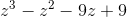.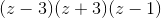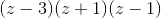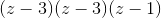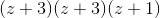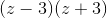Explanation:

To begin, let's factor the first two terms and the second two terms separately.

z3 – z2 – 9z + 9 = (z3 – z2) + (–9z + 9) = z2(z – 1) – 9(z – 1)

(z – 1) can be pulled out because it appears in both terms.

z3 – z2 – 9z + 9 = (z3 – z2) + (–9z + 9) = z2(z – 1) –  9(z – 1) = (z – 1)(z2 – 9)

(z2 – 9) is a difference of squares, so we can use the formula a2 – b2 = (a – b)(a + b).

z3 – z2 – 9z + 9 = (z3 – z2) + (–9z + 9) = z2(z – 1) – 9(z – 1)

= (z – 1)(z2 – 9)

= (z – 1)(z – 3)(z + 3)

### Example Question #1 : How To Factor An Equation

Factor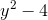.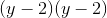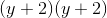None of the other answers are correct.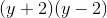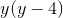Explanation:

We know the equation a2 – b2 = (a + b)(a – b) for the difference of squares. Since y2 is the square of y, and 4 is the square of 2, the correct answer is  (y + 2)(y – 2).

### Example Question #1 : How To Factor An Equation

Solve for.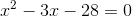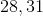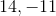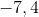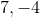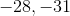Explanation:

Factor the equation by finding two numbers that add to -3 and multiply to -28.

Factors of 28: 1,2,4,7,14,28

-7 and 4 work.

(x-7)(x+4) = 0

Set each equal to zero:

x=7,-4

Tired of practice problems?

Try live online GRE Math prep today.